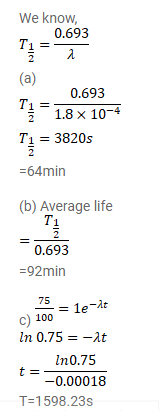# The decay constant of (electron capture to) is

Question:

The decay constant of (electron capture to) is $1.8 \times 10^{-4} \gamma^{-1}$.

(a) What is the half-life?

(b) What is the average-life?

(c) How much time will it take to convert $25 \%$ of this isotope of mercury into gold?

Solution: